# Height of the room

Given the floor area of a room as 24 feet by 48 feet and space diagonal of a room as 56 feet. Can you find the height of the room?

Correct result:

c =  16 ft

#### Solution: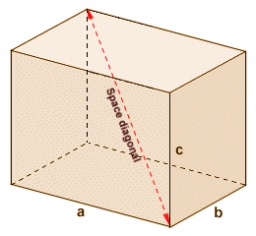We would be pleased if you find an error in the word problem, spelling mistakes, or inaccuracies and send it to us. Thank you!Tips to related online calculators
Pythagorean theorem is the base for the right triangle calculator.

#### You need to know the following knowledge to solve this word math problem:

We encourage you to watch this tutorial video on this math problem:

## Next similar math problems:

• Right triangular prismWe have cuboid with a base and dimensions of 12 cm and 5 cm and height of 4 cm. The tablecloth cut it into two identical triangular prisms with right triangular bases. The surface of the created prisms was painted with color. Calculate the surface area of
• Wall and body diagonals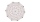Calculate the lengths of the wall and body diagonals of the cuboid with edge dimensions of 0.5 m, 1 m, and 2 m
• Faces diagonalsIf the diagonals of a cuboid are x, y, and z (wall diagonals or three faces) respectively than find the volume of a cuboid. Solve for x=1.3, y=1, z=1.2
• Rhombus baseCalculate the volume and surface area of prisms whose base is a rhombus with diagonals u1 = 12 cm and u2 = 10 cm. Prism height is twice base edge length.
• Cube diagonalsDetermine the volume and surface area of the cube if you know the length of the body diagonal u = 216 cm.
• Four sided prismCalculate the volume and surface area of a regular quadrangular prism whose height is 28.6cm and the body diagonal forms a 50 degree angle with the base plane.
• Deviation of the linesFind the deviation of the lines AG, BH in the ABCDEFGH box-cuboid, if given | AB | = 3cm, | AD | = 2cm, | AE | = 4cm
• Cuboidal room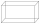Length of cuboidal room is 2m breadth of cuboidal room is 3m and height is 6m find the length of the longest rod that can be fitted in the room
• DiagonalDetermine the dimensions of the cuboid, if diagonal long 53 dm has angle with one edge 42° and with other edge 64°.
• Top of the towerThe top of the tower has the shape of a regular hexagonal pyramid. The base edge has a length of 1.2 m, the pyramid height is 1.6 m. How many square meters of sheet metal is needed to cover the top of the tower if 15% extra sheet metal is needed for joint
• Prism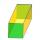The lenght, width and height of a right prism are 17, 11 and 11 respectively. What is the lenght of the longest segment whose endpoints are vertices of the prism?
• 3s prismIt is given a regular perpendicular triangular prism with a height 19.0 cm and a base edge length 7.1 cm. Calculate the volume of the prism.
• Tangent spheresA sphere with a radius of 1 m is placed in the corner of the room. What is the largest sphere size that fits into the corner behind it? Additional info: Two spheres are placed in a corner of a room. The spheres are each tangent to the walls and floor and
• Regular hexagonal prismCalculate the volume of a regular hexagonal prism whose body diagonals are 24cm and 25cm long.
• Support colum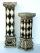Calculate the volume and surface of the support column that is shaped as perpendicular quadrangular prism whose base is a rhombus with a diagonals u1 = 102 cm u2 = 64 cm. Column height is 1. 5m.
• Distance of pointsA regular quadrilateral pyramid ABCDV is given, in which edge AB = a = 4 cm and height v = 8 cm. Let S be the center of the CV. Find the distance of points A and S.
• Ratio of edgesThe dimensions of the cuboid are in a ratio 3: 1: 2. The body diagonal has a length of 28 cm. Find the volume of a cuboid.